Скачать презентацию Integrating Topics and Syntax -Thomas L Griffiths Mark

e2e8ff86c6fa3537f429eb6af71161fb.ppt

• Количество слайдов: 31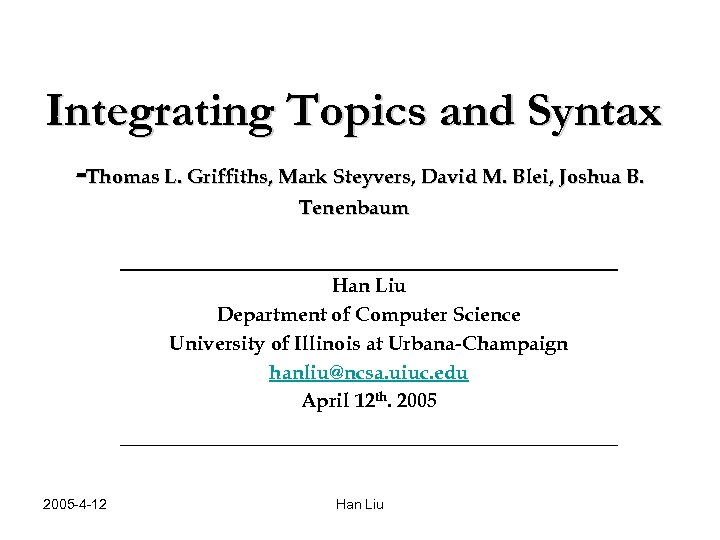Integrating Topics and Syntax -Thomas L. Griffiths, Mark Steyvers, David M. Blei, Joshua B. Tenenbaum Han Liu Department of Computer Science University of Illinois at Urbana-Champaign [email protected] uiuc. edu April 12 th. 2005 -4 -12 Han Liu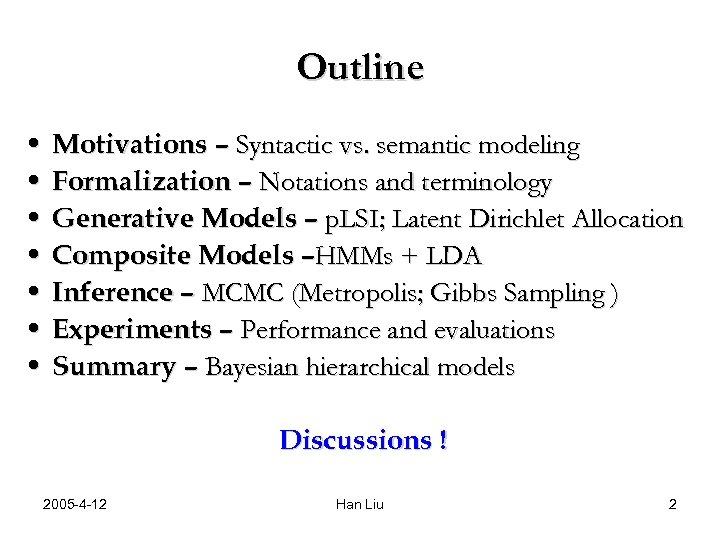Outline • Motivations – Syntactic vs. semantic modeling • Formalization – Notations and terminology • Generative Models – p. LSI; Latent Dirichlet Allocation • Composite Models –HMMs + LDA • Inference – MCMC (Metropolis; Gibbs Sampling ) • Experiments – Performance and evaluations • Summary – Bayesian hierarchical models Discussions ! 2005 -4 -12 Han Liu 2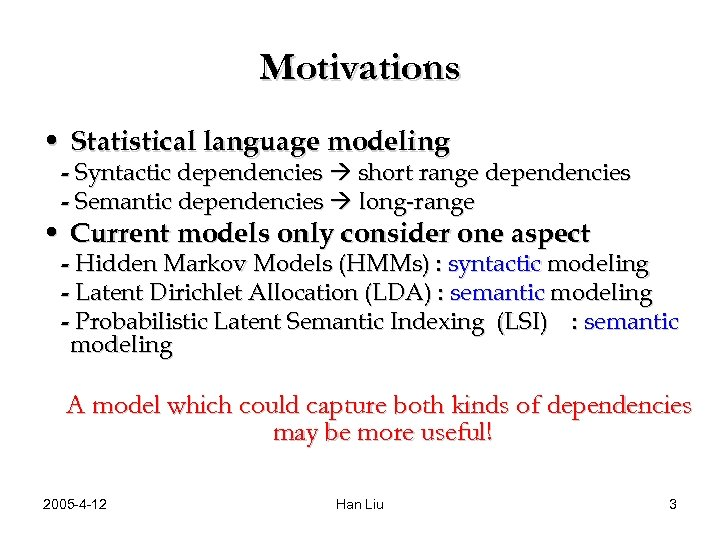Motivations • Statistical language modeling - Syntactic dependencies short range dependencies - Semantic dependencies long-range • Current models only consider one aspect - Hidden Markov Models (HMMs) : syntactic modeling - Latent Dirichlet Allocation (LDA) : semantic modeling - Probabilistic Latent Semantic Indexing (LSI) : semantic modeling A model which could capture both kinds of dependencies may be more useful! 2005 -4 -12 Han Liu 3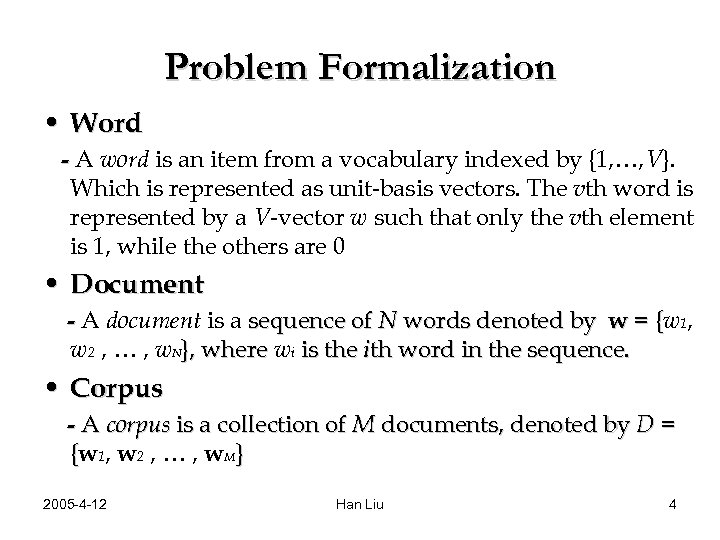Problem Formalization • Word - A word is an item from a vocabulary indexed by {1, …, V}. Which is represented as unit-basis vectors. The vth word is represented by a V-vector w such that only the vth element is 1, while the others are 0 • Document - A document is a sequence of N words denoted by w = {w 1, { w 2 , … , w. N}, where wi is the ith word in the sequence. • Corpus - A corpus is a collection of M documents, denoted by D = {w 1, w 2 , … , w. M} 2005 -4 -12 Han Liu 4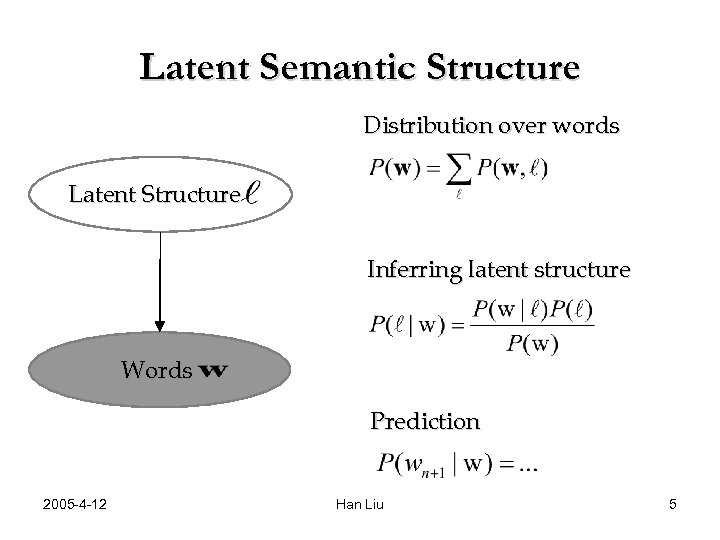Latent Semantic Structure Distribution over words Latent Structure Inferring latent structure Words Prediction 2005 -4 -12 Han Liu 5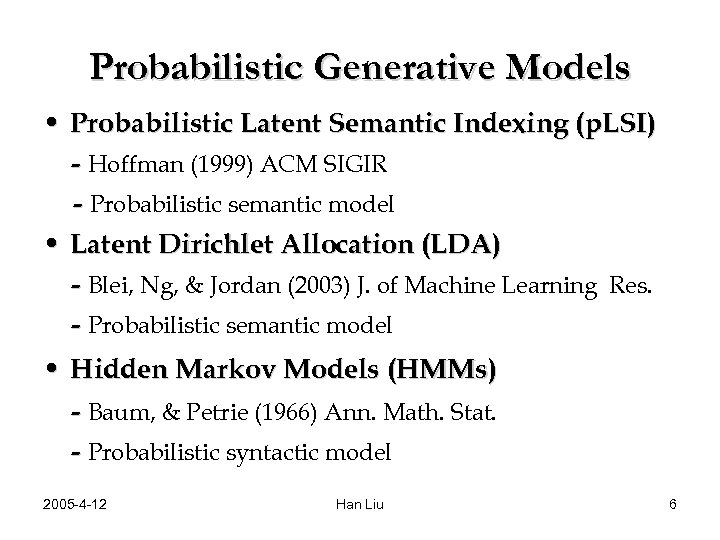Probabilistic Generative Models • Probabilistic Latent Semantic Indexing (p. LSI) - Hoffman (1999) ACM SIGIR - Probabilistic semantic model • Latent Dirichlet Allocation (LDA) - Blei, Ng, & Jordan (2003) J. of Machine Learning Res. - Probabilistic semantic model • Hidden Markov Models (HMMs) - Baum, & Petrie (1966) Ann. Math. Stat. - Probabilistic syntactic model 2005 -4 -12 Han Liu 6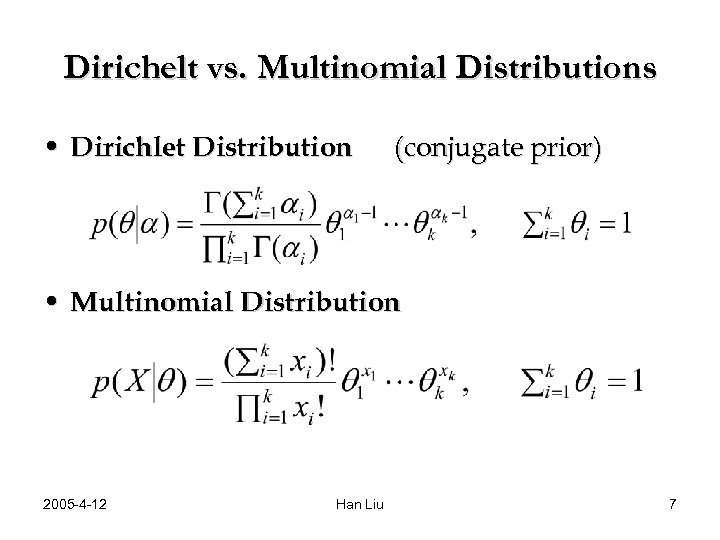Dirichelt vs. Multinomial Distributions • Dirichlet Distribution (conjugate prior) • Multinomial Distribution 2005 -4 -12 Han Liu 7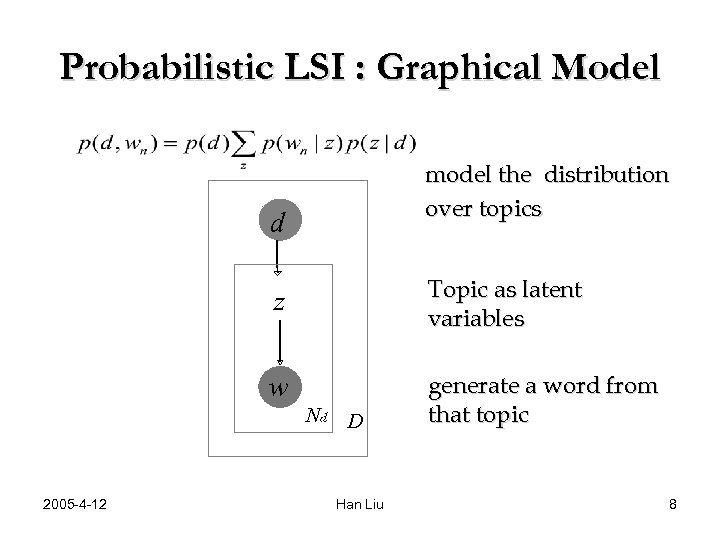Probabilistic LSI : Graphical Model model the distribution over topics d z Topic as latent variables w generate a word from that topic Nd D d 2005 -4 -12 Han Liu 8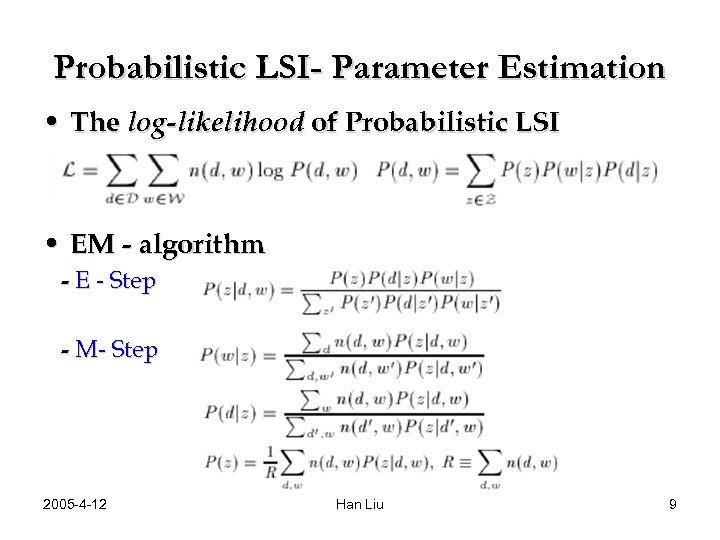Probabilistic LSI- Parameter Estimation • The log-likelihood of Probabilistic LSI • EM - algorithm - E - Step - M- Step 2005 -4 -12 Han Liu 9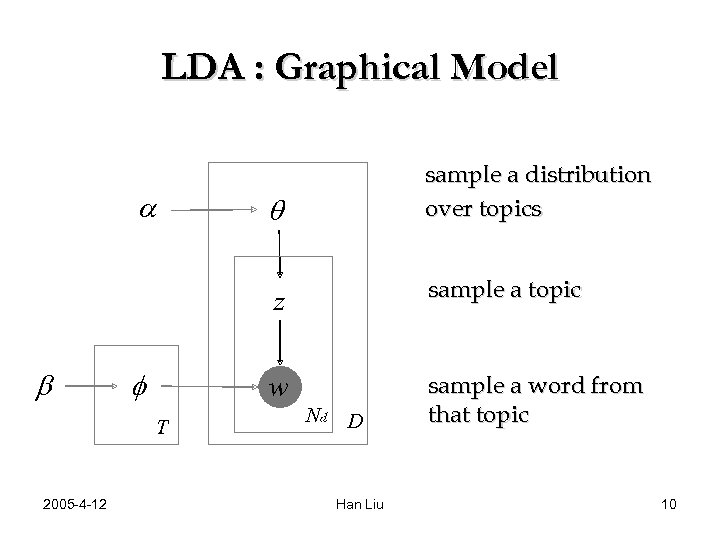LDA : Graphical Model q z b f T 2005 -4 -12 sample a topic w a sample a distribution over topics sample a word from that topic Nd D d Han Liu 10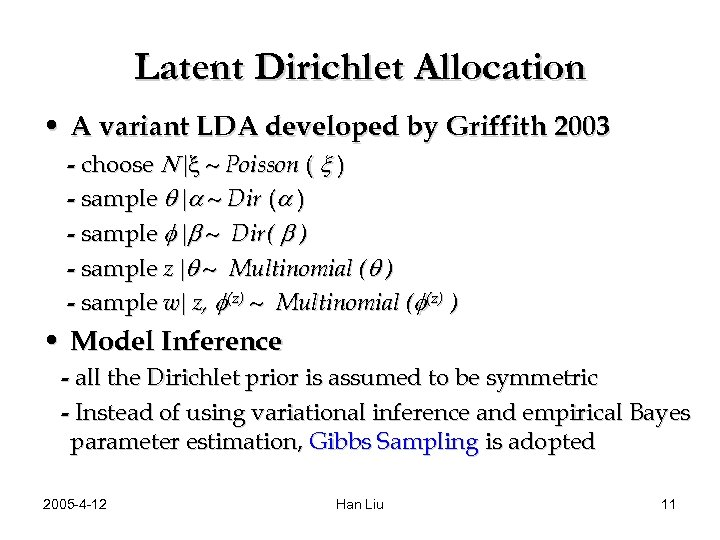Latent Dirichlet Allocation • A variant LDA developed by Griffith 2003 - choose N |x ~ Poisson ( x ) - sample q |a ~ Dir (a ) - sample f |b ~ Dir( b ) - sample z |q ~ Multinomial (q ) - sample w| z, f(z) ~ Multinomial (f(z) ) • Model Inference - all the Dirichlet prior is assumed to be symmetric - Instead of using variational inference and empirical Bayes parameter estimation, Gibbs Sampling is adopted 2005 -4 -12 Han Liu 11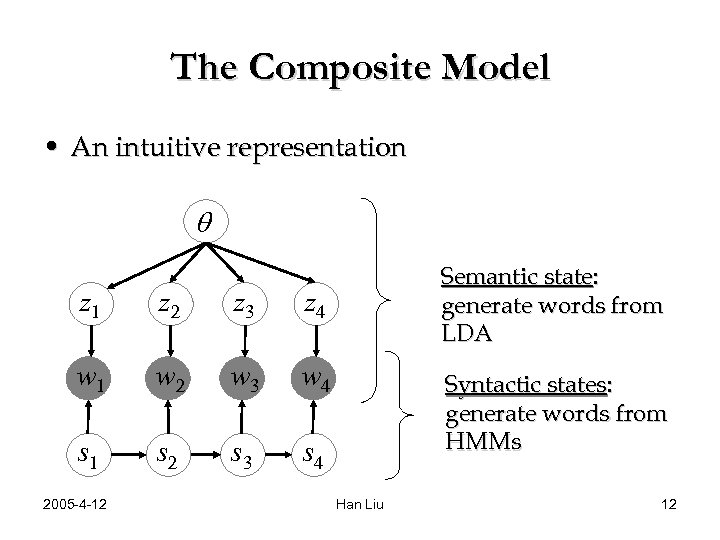The Composite Model • An intuitive representation q z 1 z 2 z 3 z 4 w 1 w 2 w 3 w 4 s 1 s 2 s 3 Semantic state: generate words from LDA s 4 2005 -4 -12 Syntactic states: generate words from HMMs Han Liu 12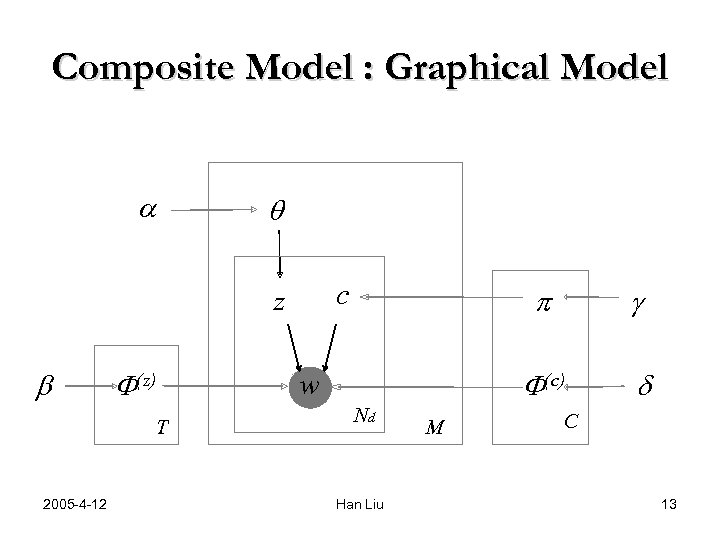Composite Model : Graphical Model a q c z b F(z) T 2005 -4 -12 p F(c) w d Nd Han Liu M g d C 13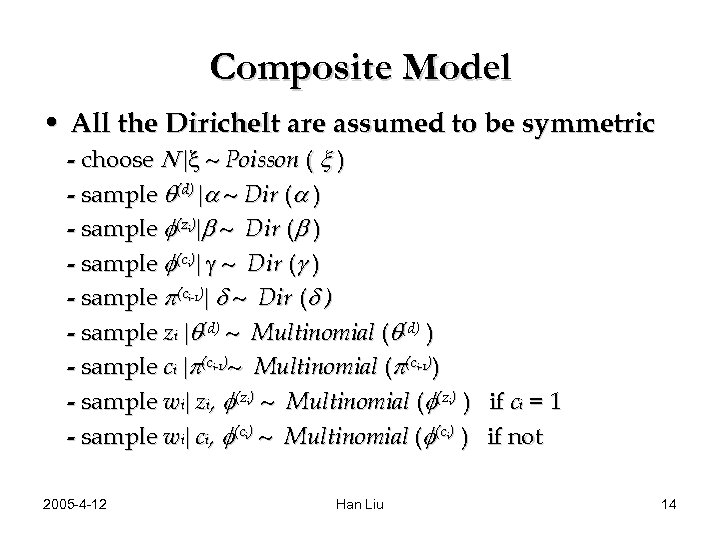Composite Model • All the Dirichelt are assumed to be symmetric - choose N |x ~ Poisson ( x ) - sample q(d) |a ~ Dir (a ) - sample f(z )|b ~ Dir (b ) - sample f(c )| g ~ Dir (g ) - sample p(c )| d ~ Dir (d ) - sample zi |q(d) ~ Multinomial (q(d) ) - sample ci |p(c )~ Multinomial (p(c )) - sample wi| zi, f(z ) ~ Multinomial (f(z ) ) if ci = 1 - sample wi| ci, f(c ) ~ Multinomial (f(c ) ) if not i i i-1 i-1 i i 2005 -4 -12 i i Han Liu 14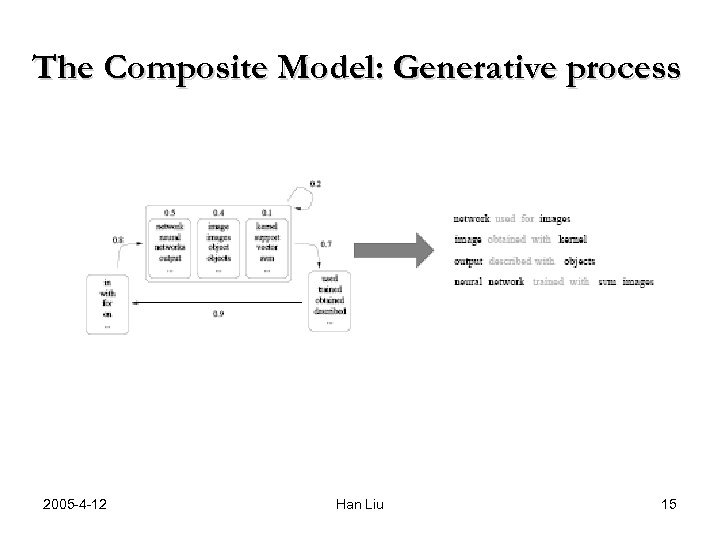The Composite Model: Generative process 2005 -4 -12 Han Liu 15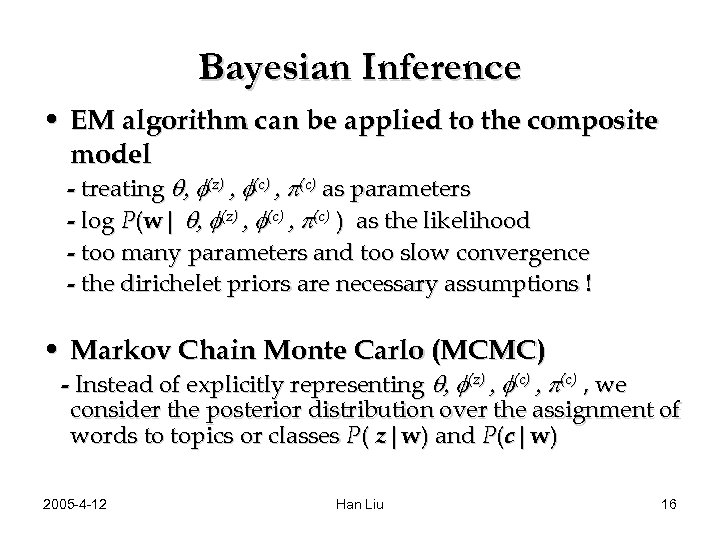Bayesian Inference • EM algorithm can be applied to the composite model - treating q, f(z) , f(c) , p(c) as parameters - log P(w| q, f(z) , f(c) , p(c) ) as the likelihood - too many parameters and too slow convergence - the dirichelet priors are necessary assumptions ! • Markov Chain Monte Carlo (MCMC) - Instead of explicitly representing q, f(z) , f(c) , p(c) , we consider the posterior distribution over the assignment of words to topics or classes P( z|w) and P(c|w) 2005 -4 -12 Han Liu 16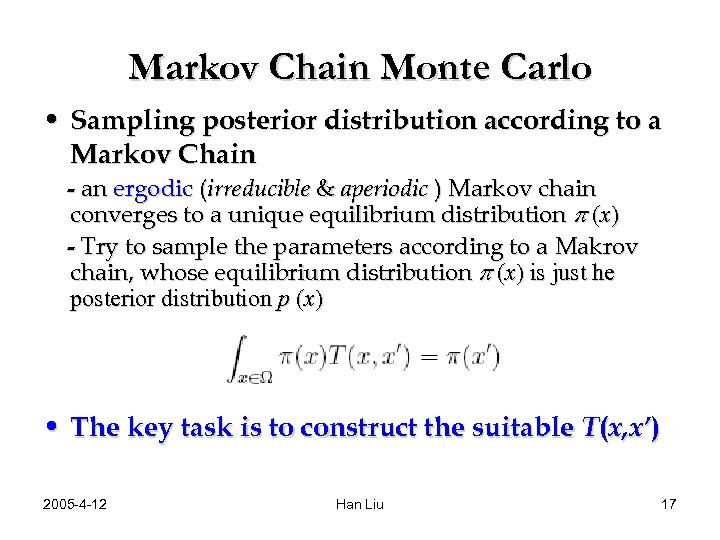Markov Chain Monte Carlo • Sampling posterior distribution according to a Markov Chain - an ergodic (irreducible & aperiodic ) Markov chain converges to a unique equilibrium distribution p (x) - Try to sample the parameters according to a Makrov chain, whose equilibrium distribution p (x) is just he posterior distribution p (x) • The key task is to construct the suitable T(x, x’) 2005 -4 -12 Han Liu 17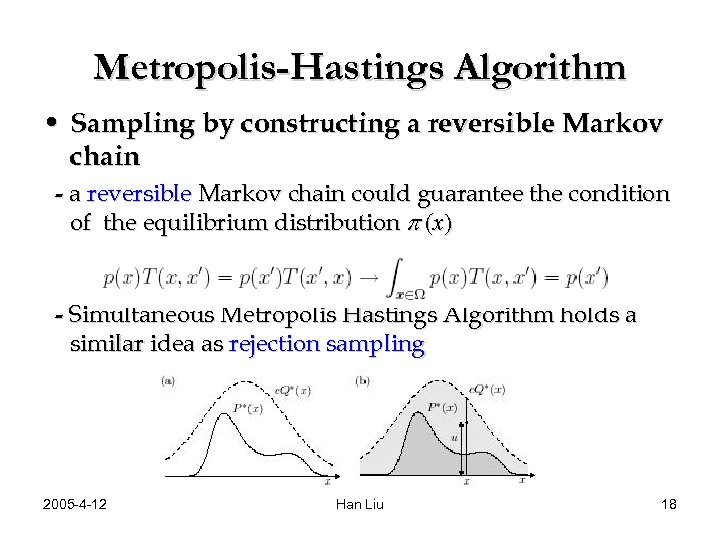Metropolis-Hastings Algorithm • Sampling by constructing a reversible Markov chain - a reversible Markov chain could guarantee the condition of the equilibrium distribution p (x) - Simultaneous Metropolis Hastings Algorithm holds a similar idea as rejection sampling 2005 -4 -12 Han Liu 18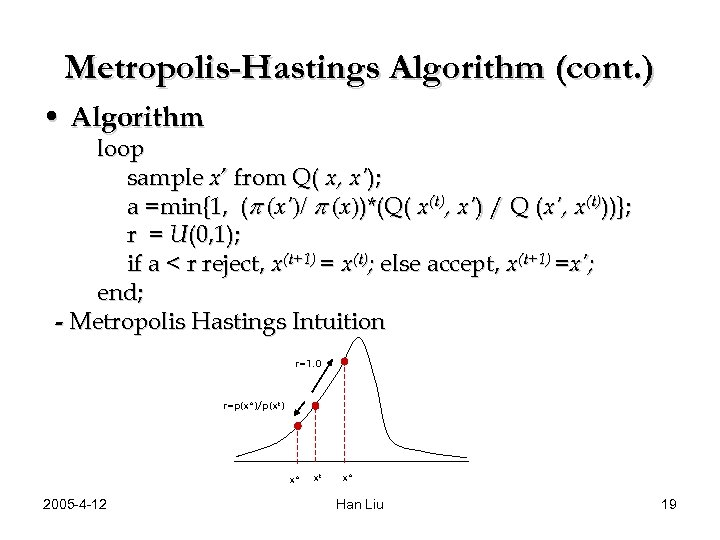Metropolis-Hastings Algorithm (cont. ) • Algorithm loop sample x’ from Q( x, x’); a =min{1, (p (x’)/ p (x))*(Q( x(t), x’) / Q (x’, x(t)))}; r = U(0, 1); if a < r reject, x(t+1) = x(t); else accept, x(t+1) =x’; end; - Metropolis Hastings Intuition r=1. 0 r=p(x*)/p(xt) x* 2005 -4 -12 xt x* Han Liu 19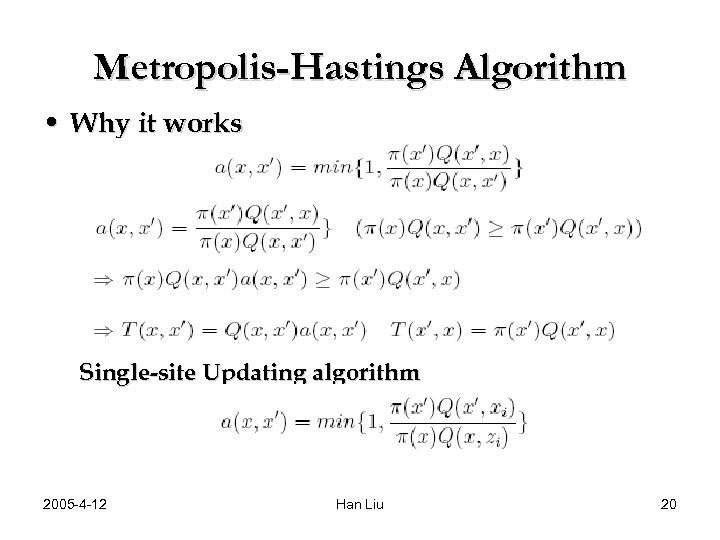Metropolis-Hastings Algorithm • Why it works Single-site Updating algorithm 2005 -4 -12 Han Liu 20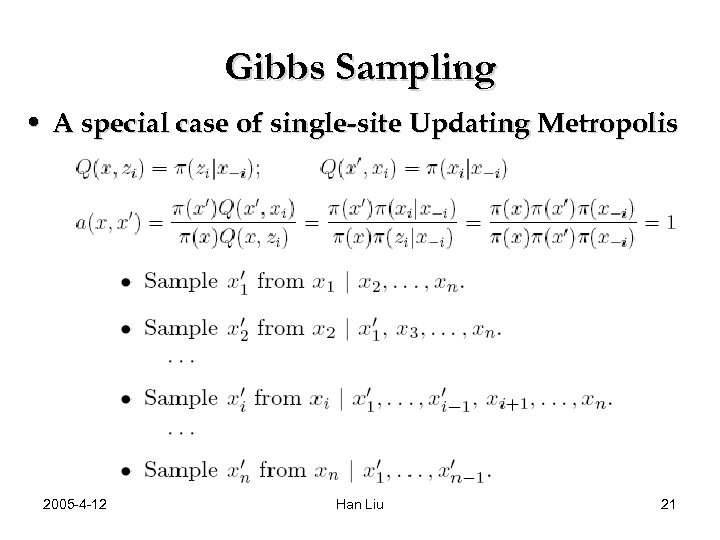Gibbs Sampling • A special case of single-site Updating Metropolis 2005 -4 -12 Han Liu 21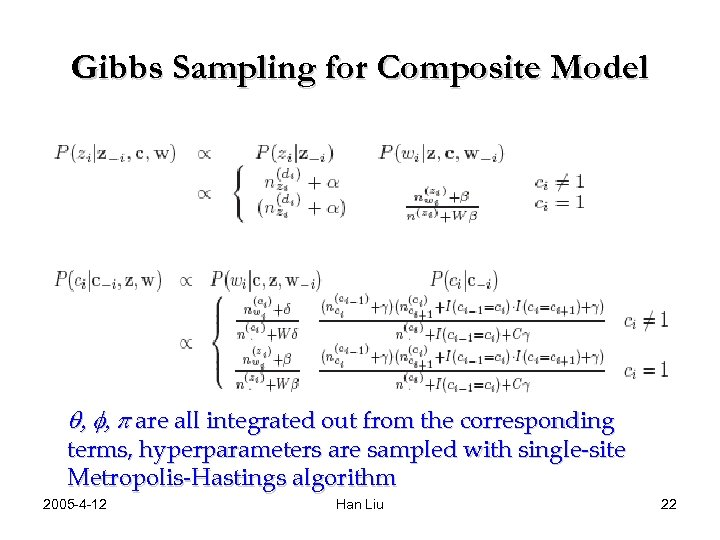Gibbs Sampling for Composite Model q, f, p are all integrated out from the corresponding terms, hyperparameters are sampled with single-site Metropolis-Hastings algorithm 2005 -4 -12 Han Liu 22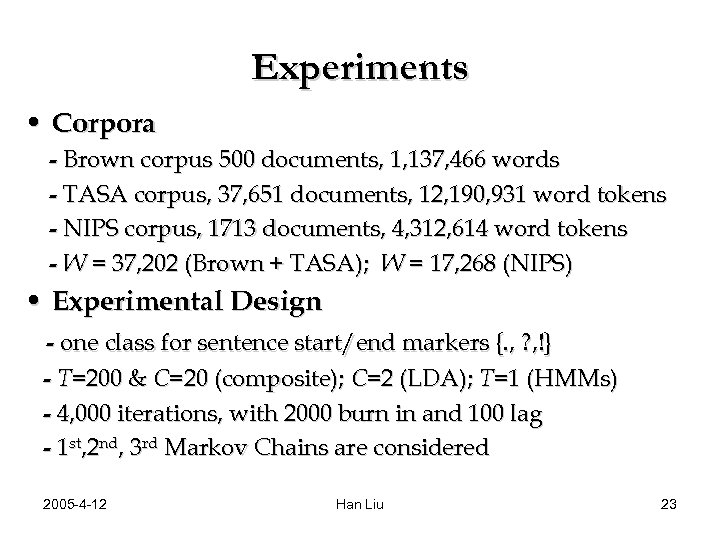Experiments • Corpora - Brown corpus 500 documents, 1, 137, 466 words - TASA corpus, 37, 651 documents, 12, 190, 931 word tokens - NIPS corpus, 1713 documents, 4, 312, 614 word tokens - W = 37, 202 (Brown + TASA); W = 17, 268 (NIPS) • Experimental Design - one class for sentence start/end markers {. , ? , !} - T=200 & C=20 (composite); C=2 (LDA); T=1 (HMMs) - 4, 000 iterations, with 2000 burn in and 100 lag - 1 st, 2 nd, 3 rd Markov Chains are considered 2005 -4 -12 Han Liu 23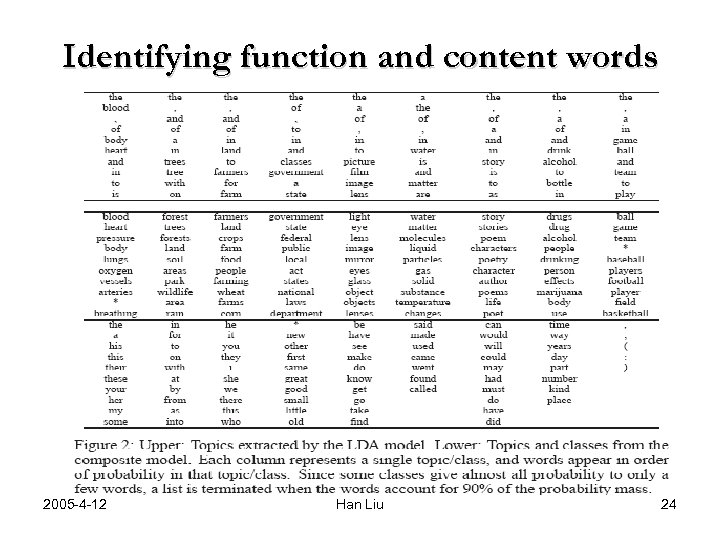Identifying function and content words 2005 -4 -12 Han Liu 24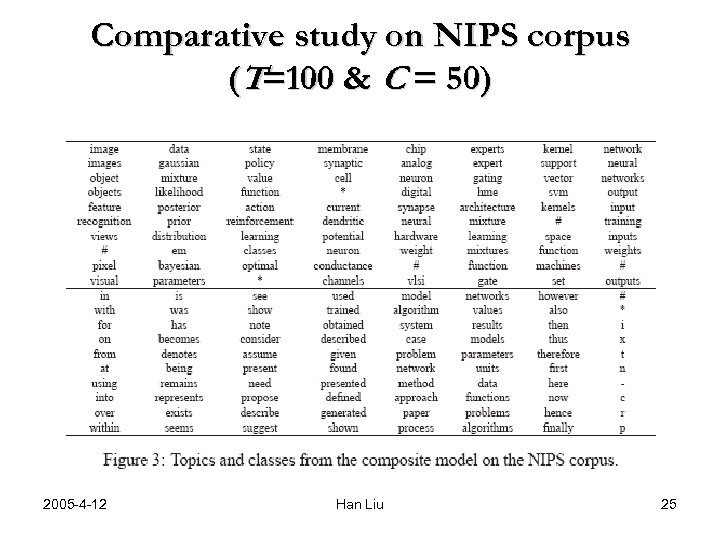Comparative study on NIPS corpus (T=100 & C = 50) 2005 -4 -12 Han Liu 25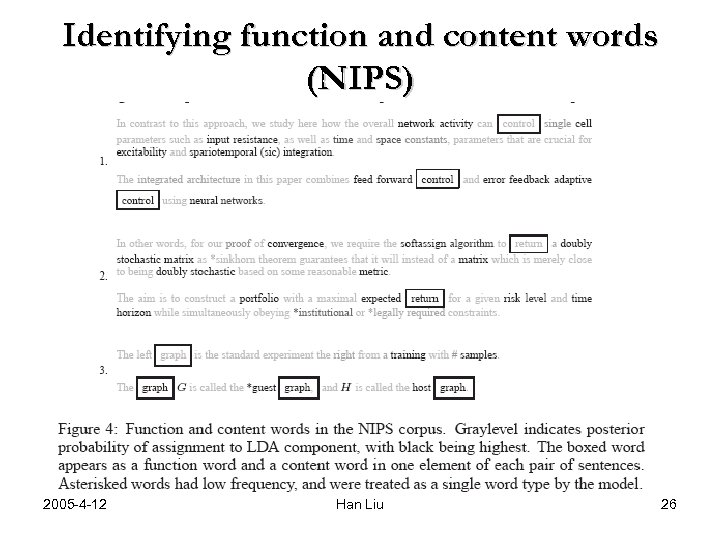Identifying function and content words (NIPS) 2005 -4 -12 Han Liu 26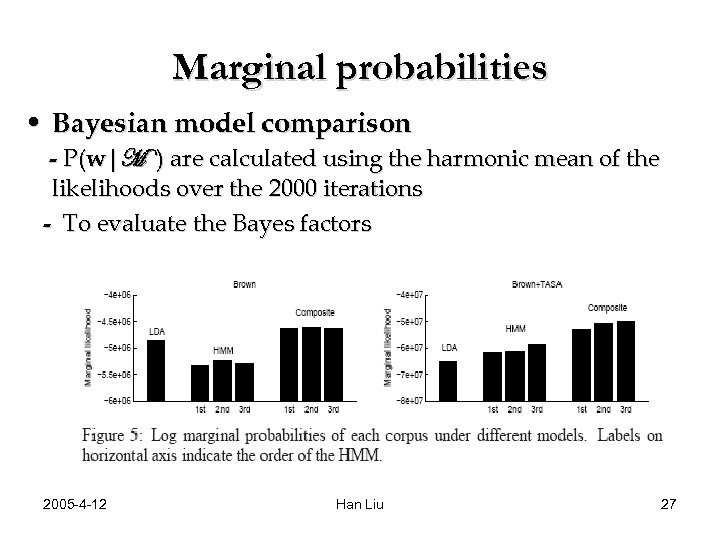Marginal probabilities • Bayesian model comparison - P(w|M ) are calculated using the harmonic mean of the likelihoods over the 2000 iterations - To evaluate the Bayes factors 2005 -4 -12 Han Liu 27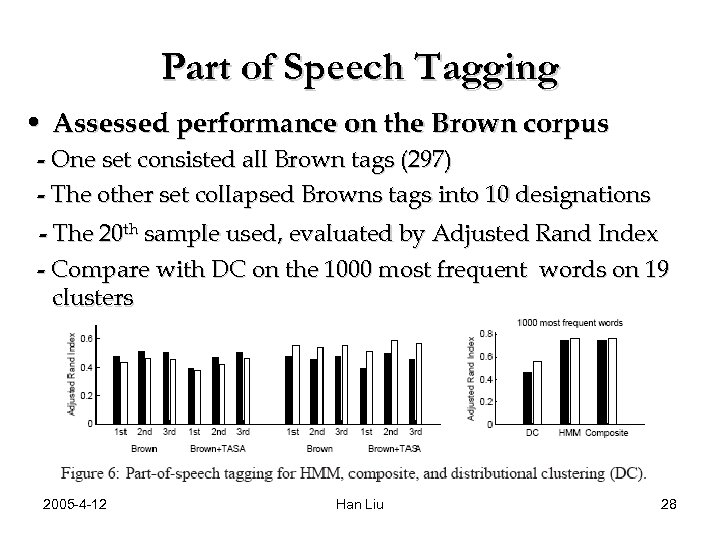Part of Speech Tagging • Assessed performance on the Brown corpus - One set consisted all Brown tags (297) - The other set collapsed Browns tags into 10 designations - The 20 th sample used, evaluated by Adjusted Rand Index - Compare with DC on the 1000 most frequent words on 19 clusters 2005 -4 -12 Han Liu 28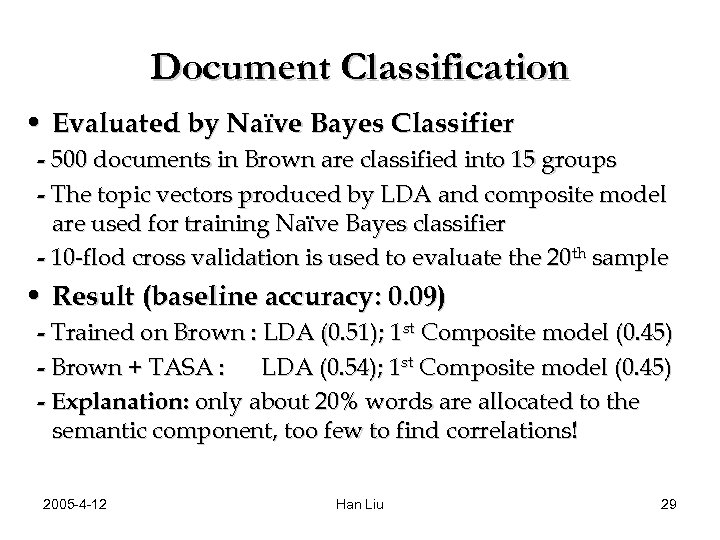Document Classification • Evaluated by Naïve Bayes Classifier - 500 documents in Brown are classified into 15 groups - The topic vectors produced by LDA and composite model are used for training Naïve Bayes classifier - 10 -flod cross validation is used to evaluate the 20 th sample • Result (baseline accuracy: 0. 09) - Trained on Brown : LDA (0. 51); 1 st Composite model (0. 45) - Brown + TASA : LDA (0. 54); 1 st Composite model (0. 45) - Explanation: only about 20% words are allocated to the semantic component, too few to find correlations! 2005 -4 -12 Han Liu 29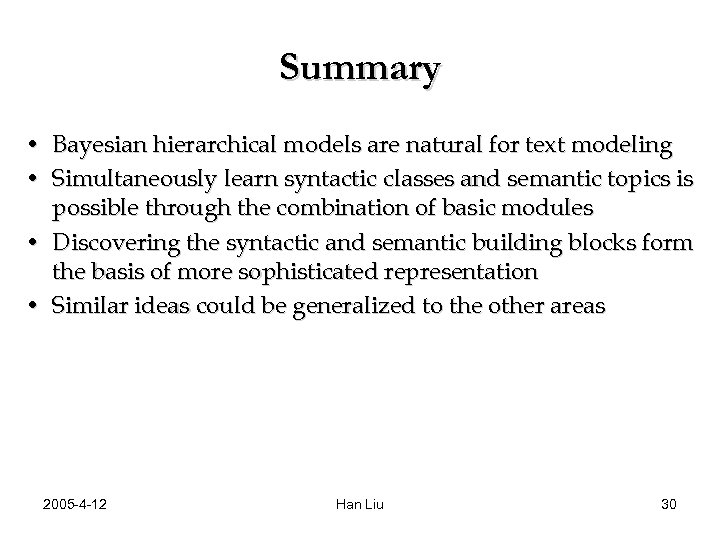Summary • Bayesian hierarchical models are natural for text modeling • Simultaneously learn syntactic classes and semantic topics is possible through the combination of basic modules • Discovering the syntactic and semantic building blocks form the basis of more sophisticated representation • Similar ideas could be generalized to the other areas 2005 -4 -12 Han Liu 30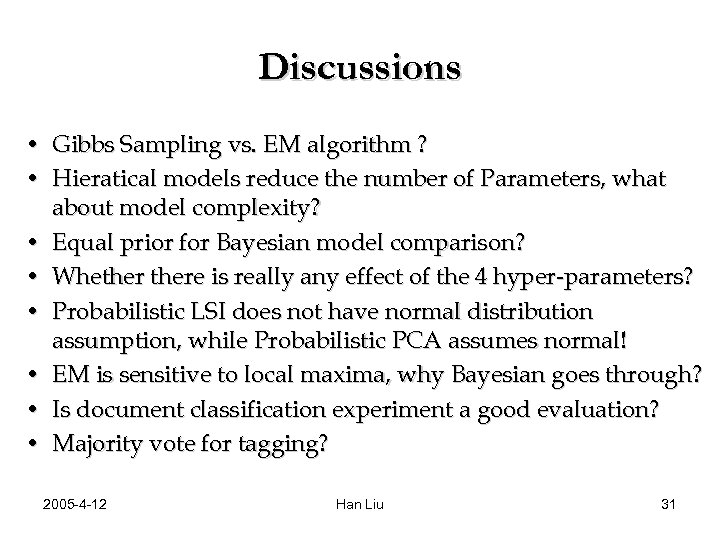Discussions • Gibbs Sampling vs. EM algorithm ? • Hieratical models reduce the number of Parameters, what about model complexity? • Equal prior for Bayesian model comparison? • Whethere is really any effect of the 4 hyper-parameters? • Probabilistic LSI does not have normal distribution assumption, while Probabilistic PCA assumes normal! • EM is sensitive to local maxima, why Bayesian goes through? • Is document classification experiment a good evaluation? • Majority vote for tagging? 2005 -4 -12 Han Liu 31